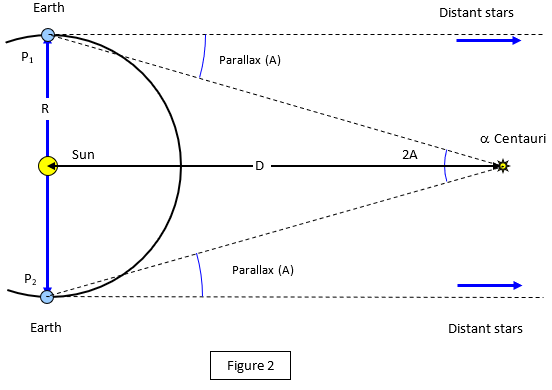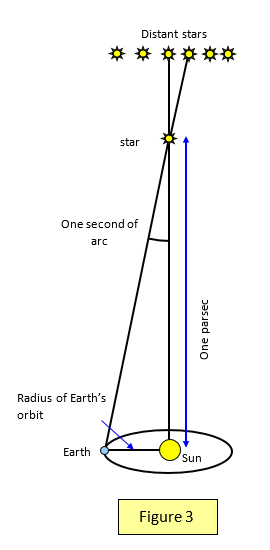# Distance measurement in Astronomy

Being able to measure the distances of astronomical objects is very important in our understanding of the scale of the Universe.

The distance from the Earth of objects in the Solar System can be measured using radar. A pulse is sent out and the time taken for the reflected pulse to be received is recorded. Knowing the speed of radar in free space and the time between transmission and reception the radar pulse enables us to find the distance of the object.

For example the time between the radar pulse being sent out and the reflected pulse being received would be 2.5 s for the Moon and up to 50 minutes for Jupiter and around five and a half hours for Pluto. (The last two numbers depend on the relative positions of the Earth, Jupiter and Pluto in their orbits)

For objects outside the solar system other methods have to be found.

## Parallax

You can find the distances of stars that are 'relatively close' to the Earth using parallax.

A very simple example of parallax is to hold one forefinger upright about 30 cm in front of your nose and close your right eye. Using just your left eye line up your forefinger with an object on the other side of the room. Now open your right eye and close your left eye. Your finger appears to move against the background.

To understand exactly what is meant by parallax go and stand at one end of the lab, you will be the observer. Now get one member of your class to stand at A in the middle of the lab (they represent a star that is close to the Earth and we will call them the 'nearby star') and some others to stand at the far end (they represent distant stars). Look the 'nearby star' and see which of the 'distant stars' lie in line with it (See Figure 1).

Now walk across the lab to Position 2 and check again which of the 'distant stars' is in line with the 'nearby star ', you will see that the apparent position of the 'nearby star' compared with the distance stars has changed.The angle between the two directions in positions 1 and 2 is 2P, and half that angle is called the PARALLAX (P) of the 'nearby star'.
Now look at the 'nearby star' when it is in position B. The parallax here is Q and you can see two things about the 'nearby star' now:
(a) it is further away from the observer
(b) the parallax of the star is smaller than that of the 'star' at A

Figure 2 shows the use of parallax to measure the distance of an actual star – say Alpha Centauri. This time the 'base line' is the diameter of the Earth's orbit – in other words a line joining the position of the Earth at two points six months apart.The direction of Alpha Centauri is measured against the background of the distant stars at the two points P1 and P2. The angle 2A is measured and so the parallax (angle A) can be found.

If you know the angle A and the radius of the Earth's orbit (R) you can find the distance of the star (D). Stars that are close to the Earth clearly have a larger parallax than ones far away.

Even nearby Stars are so far away that their direction changes only slightly against the background of the distant stars when seen from points on the Earth's orbit six months apart.

Nearby stars have a parallax of only a fraction of a second of arc (one second of arc is 1/3600 of a degree).

In the example shown Alpha Centauri (distance 4.3 light years) has a parallax of 0.75 " of arc.

A more distant star such as Spica in the constellation of Virgo, which is 220 light years away, has a parallax of only 0.023".

## The Parsec

Astronomers use the parallax of the star to define a new distance unit called the PARSEC.

1 parsec is the distance of an object which has a parallax of 1” of arc. This means that it subtends an angle of one second using the radius of the Earth’s orbit as the baseline.

The radius of Earth's orbit = 1.5x1011 m and therefore if theparallax of a star is 1" the distance can be found from:

tan(1") = 1.5x1011/d so d = 3.06 x1016 m (See Figure 3)

Distances between galaxies are usually measured in millions of parsecs or Mega parsecs (Mpc).

1 parsec = 3.06x1016 m = 2.04x105 AU = 3.26 light years
1 Mega parsec = 3.06x1022 m = 2.04x1011 AU = 3.26x106 light years

The parallax of a number of stars is shown in the following table.

 Star Parallax(" of arc) Distance (l.y) Star Parallax(" of arc) Distance (l.y) a Centauri 0.750 4.3 Vega 0.133 25 Barnard's star 0.545 6.0 Arcturus 0.097 34 Sirius 0.377 8.6 Aldebaran 0.054 60 Procyon 0.285 11.4 Adhara 0.067 570

At distances much greater than this the parallax method becomes impossibly difficult to measure. Remember that 1" of arc is the angle subtended by a human head almost ľ of a kilometre away. Therefore the parallax of Castor is the same as the angle subtended by a human head at a distance of almost 750 km!

A VERSION IN WORD IS AVAILABLE ON THE SCHOOLPHYSICS USB